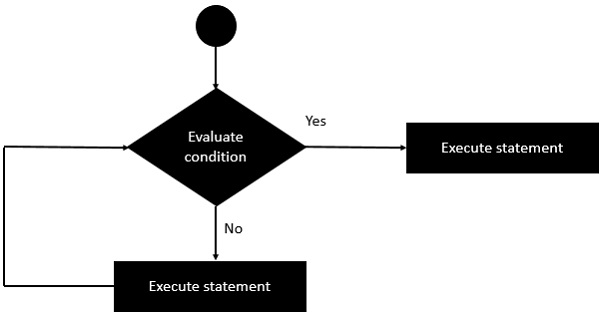# Clojure - If Statement

The first decision-making statement is the ‘if’ statement. Following is the general form of this statement in Clojure.

## Syntax

```if (condition) statement#1 statement #2
```

In Clojure, the condition is an expression which evaluates it to be either true or false. If the condition is true, then statement#1 will be executed, else statement#2 will be executed. The general working of this statement is that first a condition is evaluated in the ‘if’ statement. If the condition is true, it then executes the statements. Following diagram shows the flow of the ‘if’ statement.## Example

Following is an example of the simple ‘if’ expression in Clojure.

```(ns clojure.examples.hello
(:gen-class))

;; This program displays Hello World
(defn Example [] (
if ( = 2 2)
(println "Values are equal")
(println "Values are not equal")))
(Example)
```

## Output

The output of the above program will be “Values are equal”. In the above code example, the ‘if’ condition is used to evaluate whether the values of 2 and 2 are equal. If they are, then it will print the value of “Values are equal” else it will print the value of “Values are not equal”.

```Values are equal
```
clojure_decision_making.htm